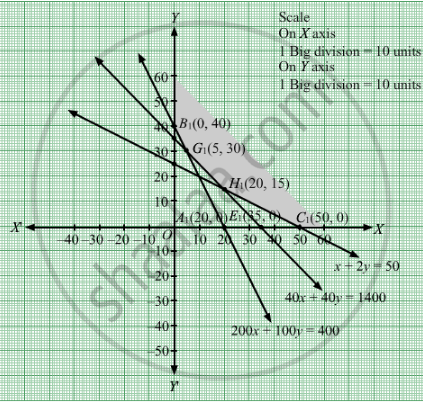# A Diet for a Sick Person Must Contain at Least 4000 Units of Vitamins, 50 Units of Minerals and 1400 of Calories. Two Foods a and B, Are Available at a Cost of Rs 4 and Rs 3 per Unit Respectively. - Mathematics

Sum

A diet for a sick person must contain at least 4000 units of vitamins, 50 units of minerals and 1400 of calories. Two foods A and B, are available at a cost of Rs 4 and Rs 3 per unit respectively. If one unit of A contains 200 units of vitamin, 1 unit of mineral and 40 calories and one unit of food B contains 100 units of vitamin, 2 units of minerals and 40 calories, find what combination of foods should be used to have the least cost?

#### Solution

Let the sick person takes x units and y units of food I and II respectively that were taken in the diet.
Since, per unit of food I costs Rs 4 and that of food II costs Rs 3.
Therefore, x units of food I costs Rs 4x and y units of food II costs Rs 3y.
Total cost = Rs (4x + 3y)
Let Z denote the total cost
Then, Z = 4+ 3y
If one unit of A contains 200 units of vitamin and one unit of food B contains 100 units of vitamin.
Thus, x units of food I and y units of food II contains 200x + 100y units of vitamin.
But a diet for a sick person must contain at least 4000 units of vitamins.

∴  $200x + 100y \geq 4000$
If one unit of A contains 1 unit of mineral and one unit of food B contains 2 units of mineral.
Thus, x units of food I and y units of food II contains x + 2y units of mineral.

But a diet for a sick person must contain at least 50 units of vitamins.

∴  $x + 2y \geq 50$ If one unit of A contains 40 calories and one unit of food B contains 40 calories.
Thus, x units of food I and y units of food II contains 40x + 40y units of calories.

But a diet for a sick person must contain at least 1400 calories.

$\therefore 40x + 40y \geq 1400$
Finally, the quantities of food I and food II are non negative values.
So
$x, y \geq 0$
Hence, the required LPP is as follows:
Min Z = 4x + 3y
subject to
$200x + 100y \geq 4000$
$x + 2y \geq 50$
$40x + 40y \geq 1400$
$x, y \geq 0$

First, we will convert the given inequations into equations, we obtain the following equations:
200x + 100y = 4000, x +2y = 50, 40x + 40y =1400, x = 0 and y = 0

Region represented by 200x + 100y ≥ 4000:
The line 200x + 100y = 4000 meets the coordinate axes at A1(20, 0) and B1(0,40) respectively. By joining these points we obtain the line
200x + 100y = 4000.Clearly (0,0) does not satisfies the inequation 200x + 100y ≥ 4000. So,the region in xy plane which does not contain the origin represents the solution set of the inequation 200x + 100y ≥ 4000.

Region represented by x +2y ≥ 50:
The line x +2y = 50 meets the coordinate axes at C1(50, 0) and D1(0, 25) respectively. By joining these points we obtain the line
x +2y = 50.Clearly (0,0) does not satisfies the x +2y ≥ 50. So,the region which does not contains the origin represents the solution set of the inequation x +2y ≥ 50.

Region represented by 40x + 40y ≥ 1400:
The line 40x + 40y = 1400 meets the coordinate axes at E1(35, 0) and F1(0, 35) respectively. By joining these points we obtain the line
40x + 40y = 1400.Clearly (0,0) does not satisfies the inequation 40x + 40y ≥ 1400. So,the region which does not contains the origin represents the solution set of the inequation 40x + 40y ≥ 1400.

Region represented by x ≥ 0 and y ≥ 0:
Since, every point in the first quadrant satisfies these inequations. So, the first quadrant is the region represented by the inequations x ≥ 0, and ≥ 0.
The feasible region determined by the system of constraints 200x + 100y ≥ 4000,x +2y ≥ 50, 40x + 40y ≥ 1400, x ≥ 0, and y ≥ 0 are as follows.The corner points of the feasible region are B1(0, 40), G1(5, 30), H1(20, 15) and C1(50, 0)

The value of the objective function at these points are given by the following table

 Points Value of Z B1 4(0)+3(40) = 120 G1 4(5)+3(30) = 110 H1 4(20) + 3(15) = 125 C1 4(50)+3(0) = 200

The minimum cost is Rs 110 which is at G1(5, 30).

Hence, the required combination of food is 5 units of food A and 30 units of food B.

Concept: Graphical Method of Solving Linear Programming Problems
Is there an error in this question or solution?

#### APPEARS IN

RD Sharma Class 12 Maths
Chapter 30 Linear programming
Exercise 30.3 | Q 2 | Page 38